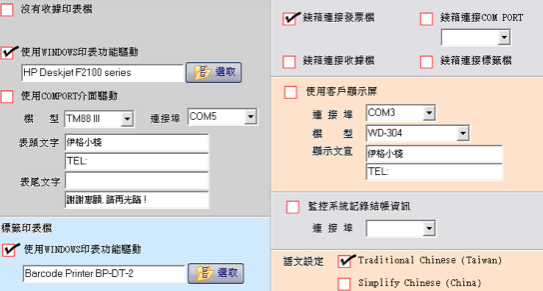## 常見的餐飲、飲料POS機硬體設定問題

• LPT 點矩陣式印表機用
• Line In/Out 麥克風/喇叭
• COM(Serial port) 主要POS機硬體規格
• USB
• LAN 網路
• PS/2 鍵盤、滑鼠
• VGA 可以第二台螢幕
• RJ11
• DC 電源## Delphi XE3 簡訊發送問題

[pascal]
function EnCode(ACode: string): string;
var
I: Integer;
Hex: string;
Code: AnsiString;
begin
Code := AnsiString(ACode);
for I := 1 to Length(Code) do
case Code[i] of
‘ ‘: Result := Result + ‘+’;
‘A’..’Z’, ‘a’..’z’, ‘*’, ‘@’, ‘.’, ‘_’, ‘-‘,
‘0’..’9′, ‘\$’, ‘!’, ””, ‘(‘, ‘)’:
Result := Result + Code[i];
else
begin
Hex := IntToHex(ord(Code[i]), 2);
if Length(Hex) = 2 then
Result := Result + ‘%’ + Hex
else
Result := Result + ‘%0’ + hex;
end;
end;
end;
[/pascal]